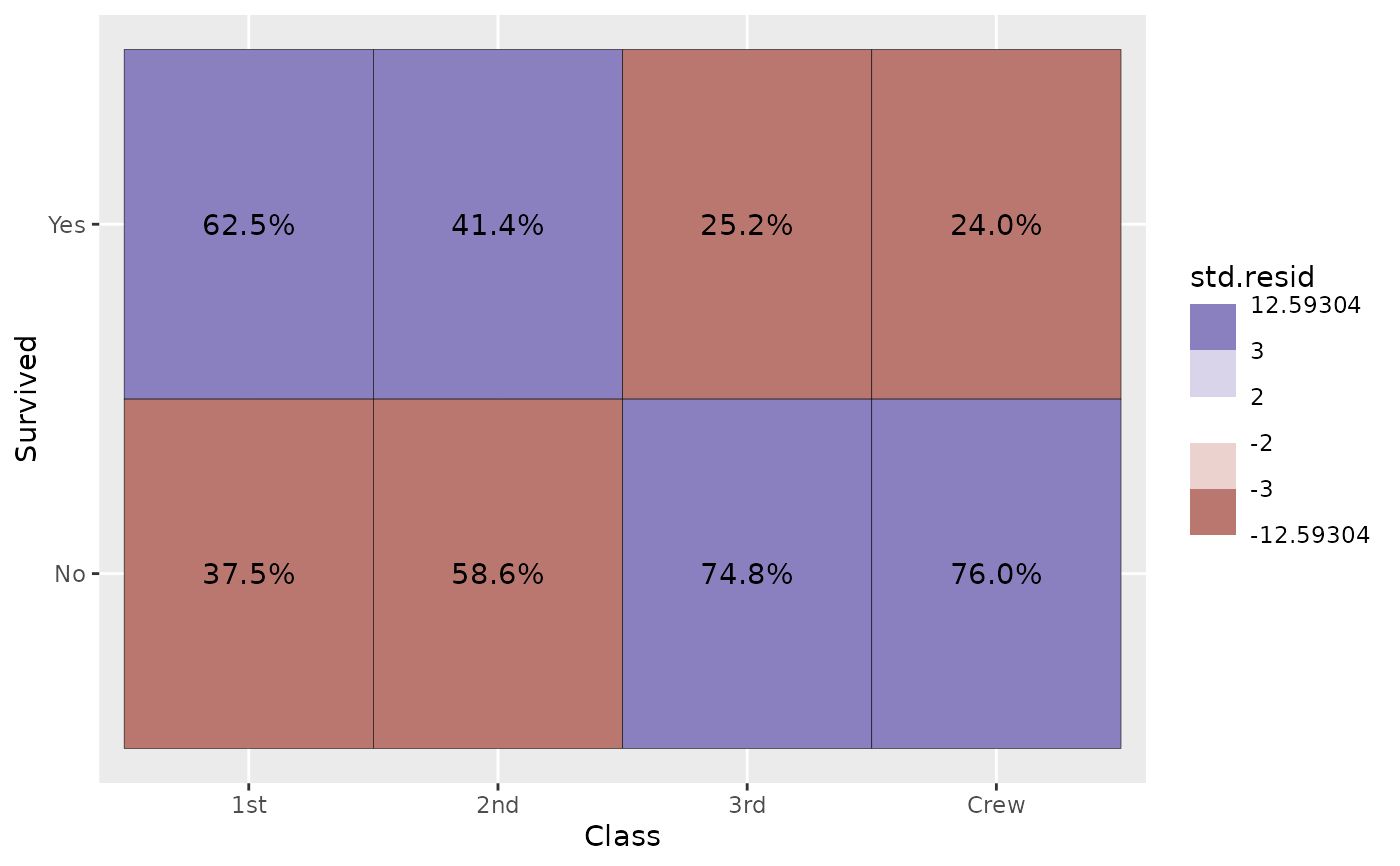Plot the number of observations as a table. Other statistics computed by stat_cross could be used (see examples).

ggally_table(
data,
mapping,
keep.zero.cells = FALSE,
...,
geom_tile_args = NULL
)

ggally_tableDiag(
data,
mapping,
keep.zero.cells = FALSE,
...,
geom_tile_args = NULL
)

## Arguments

data data set using aesthetics being used If TRUE, display cells with no observation. other arguments passed to geom_text(...) other arguments passed to geom_tile(...)

## Note

The colour aesthetic is taken into account only if equal to x or y.

## Author

Joseph Larmarange

## Examples

# Small function to display plots only if it's interactive
p_ <- GGally::print_if_interactive

data(tips, package = "reshape")
p_(ggally_table(tips, mapping = aes(x = smoker, y = sex)))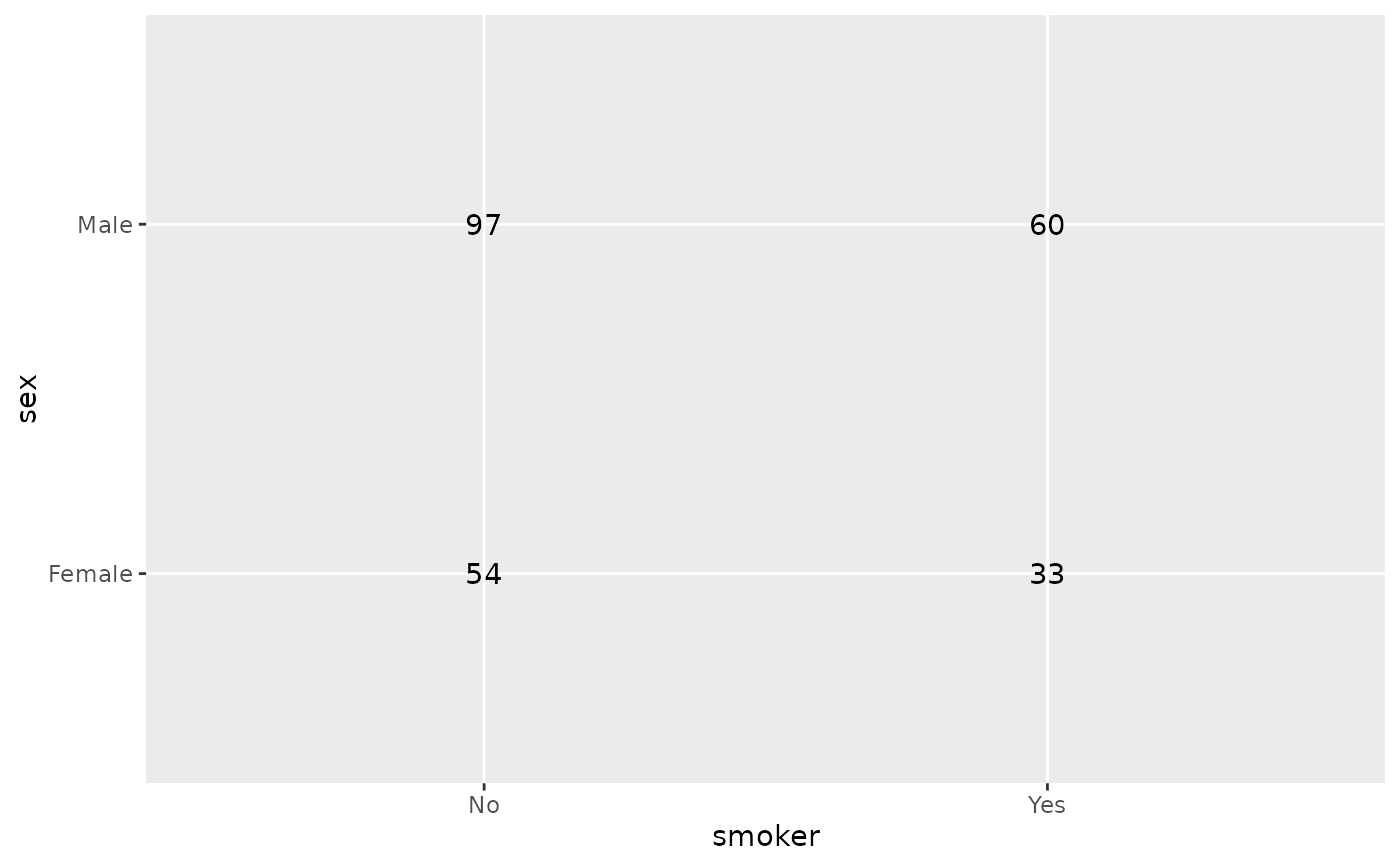p_(ggally_table(tips, mapping = aes(x = day, y = time)))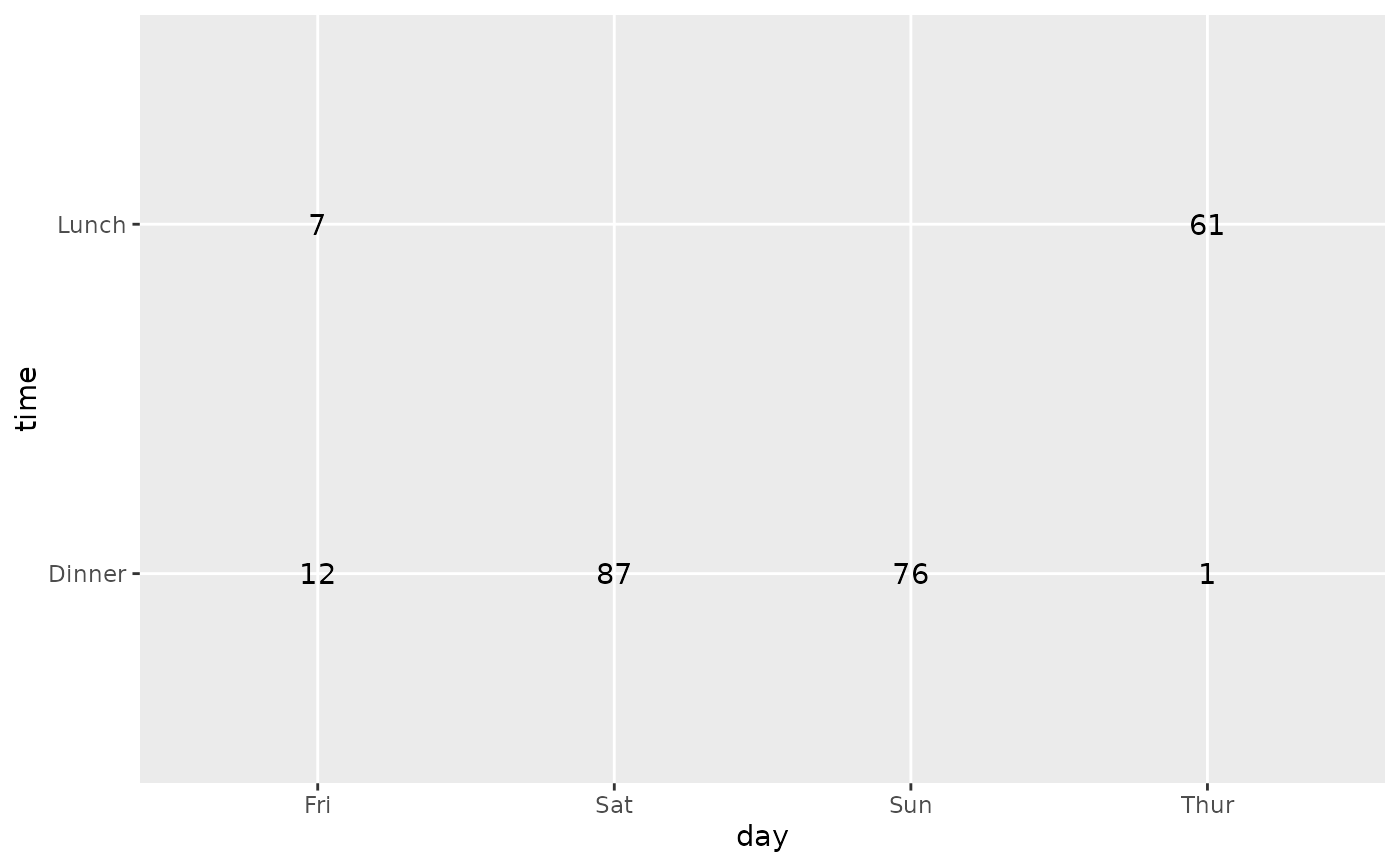p_(ggally_table(tips, mapping = aes(x = smoker, y = sex, colour = smoker)))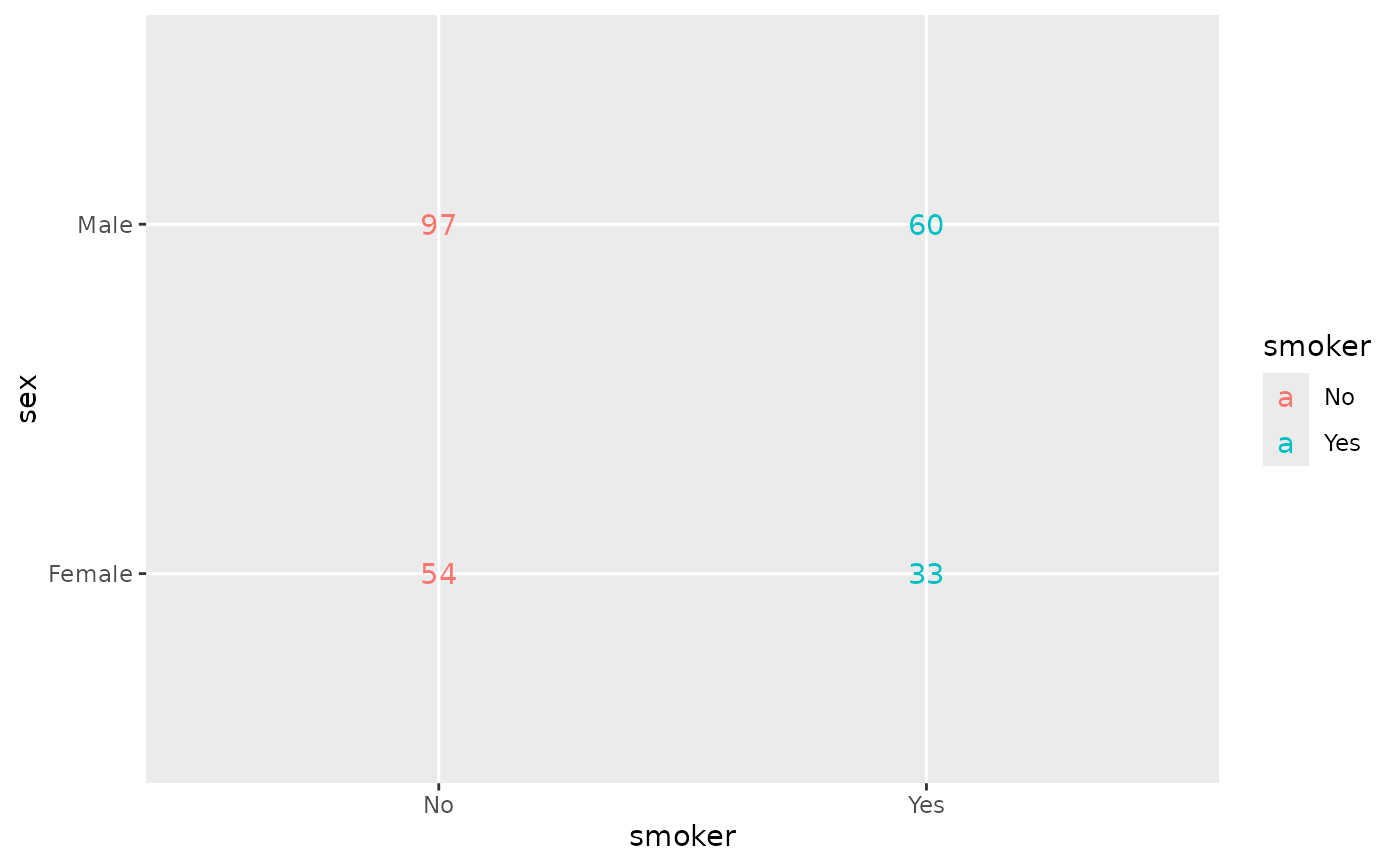# colour is kept only if equal to x or y
p_(ggally_table(tips, mapping = aes(x = smoker, y = sex, colour = day)))# diagonal version
p_(ggally_tableDiag(tips, mapping = aes(x = smoker)))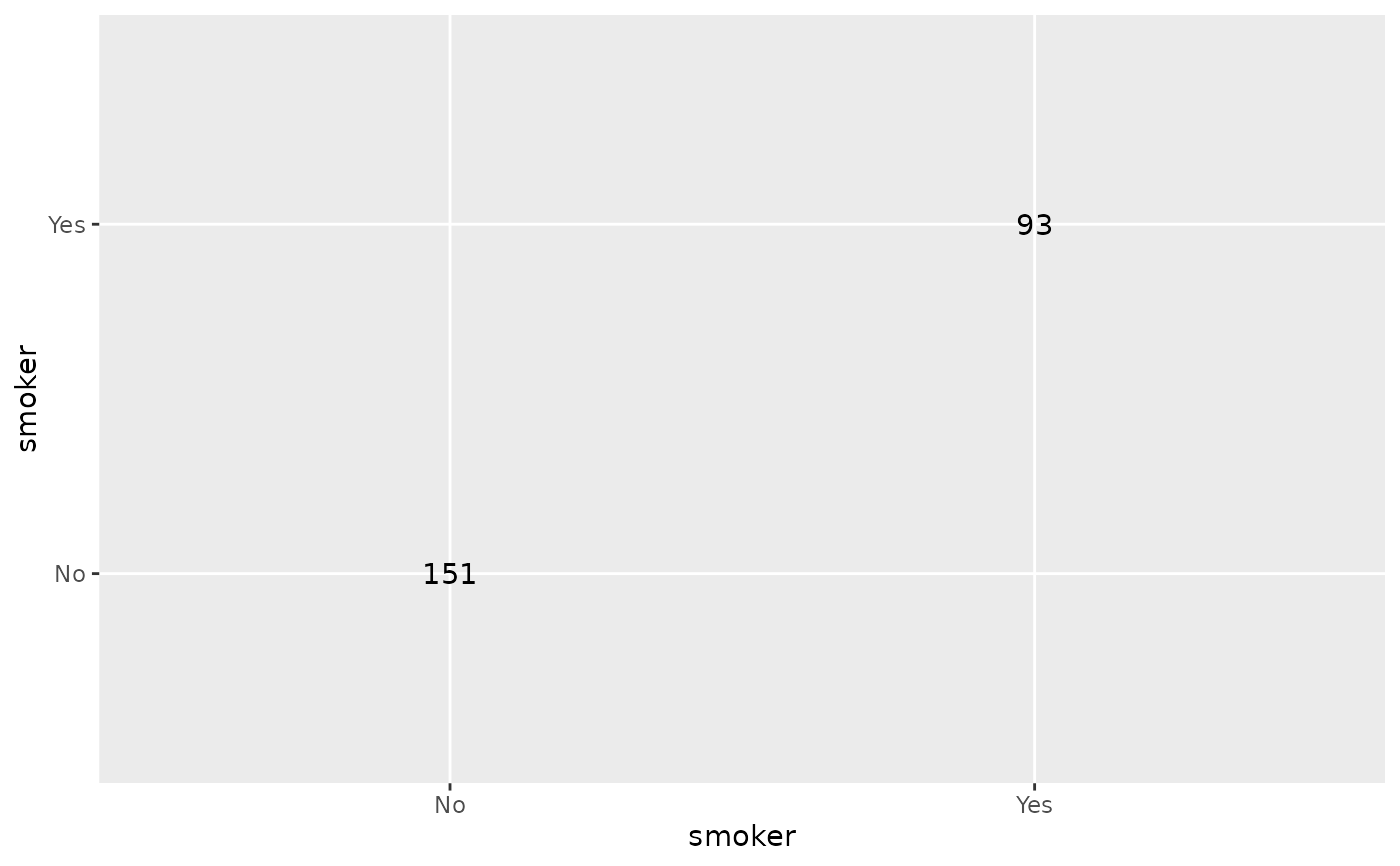# custom label size and color
p_(ggally_table(tips, mapping = aes(x = smoker, y = sex), size = 16, color = "red"))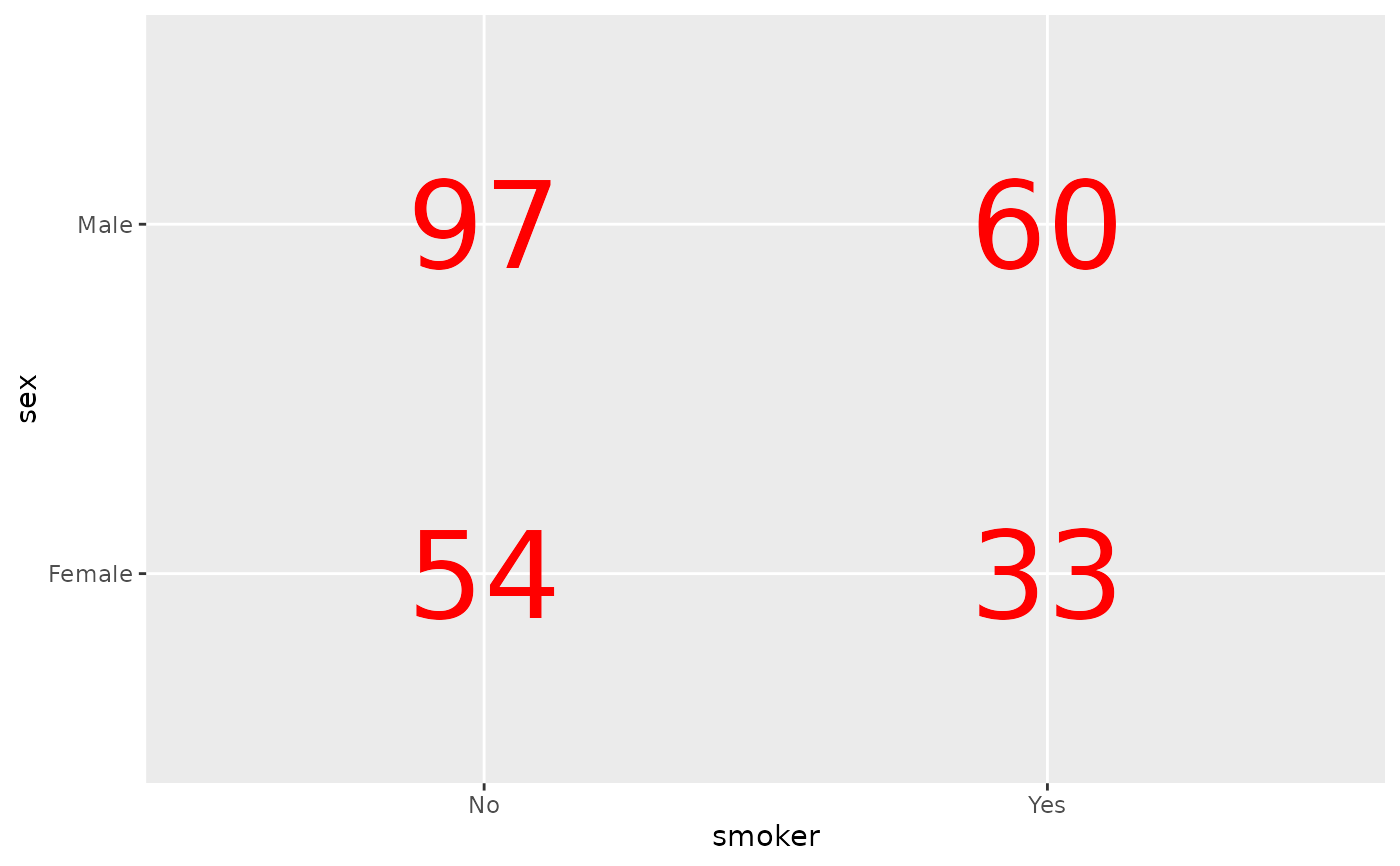# display column proportions
p_(ggally_table(
tips,
mapping = aes(x = day, y = sex, label = scales::percent(after_stat(col.prop)))
))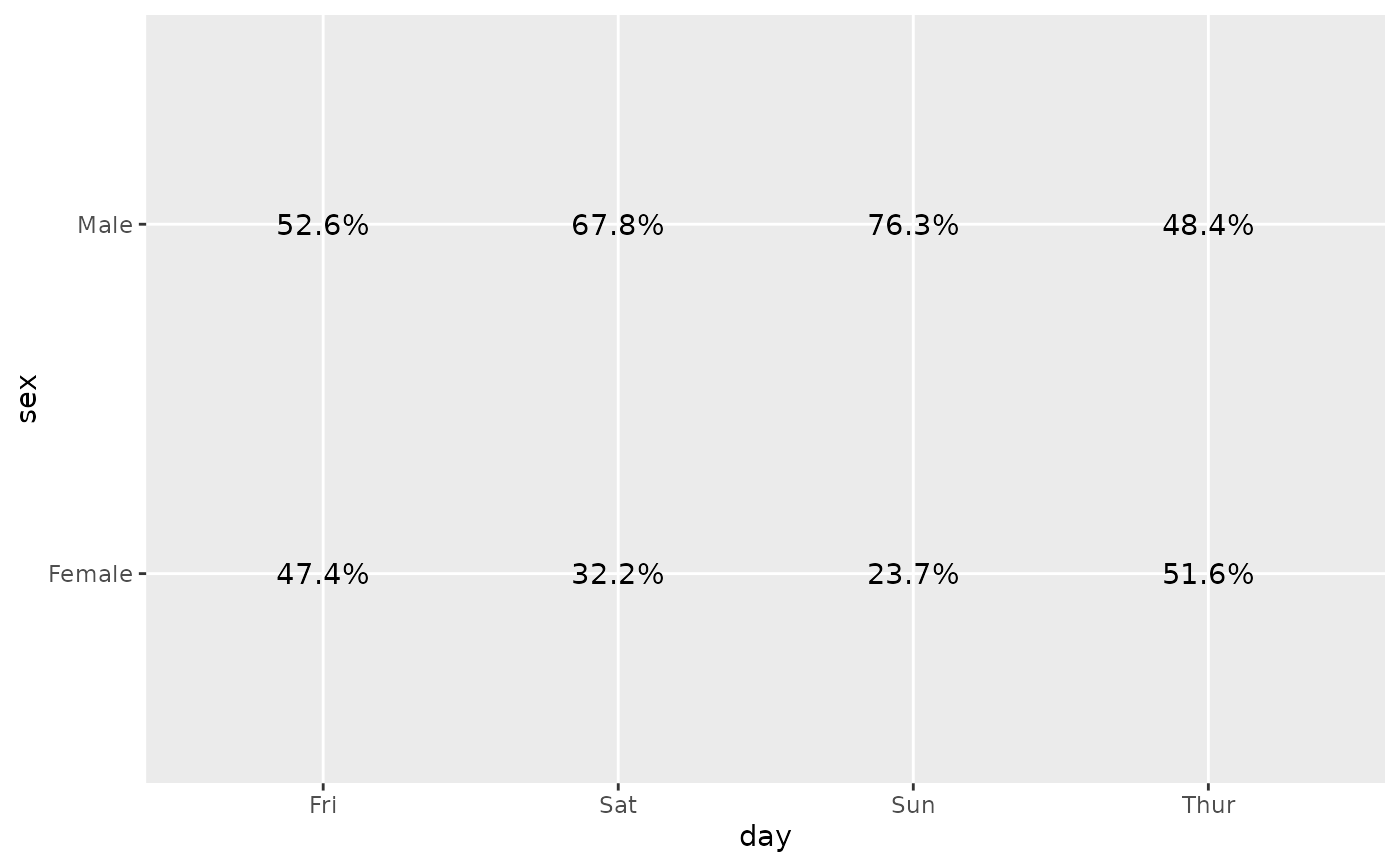# draw table cells
p_(ggally_table(
tips,
mapping = aes(x = smoker, y = sex),
geom_tile_args = list(colour = "black", fill = "white")
))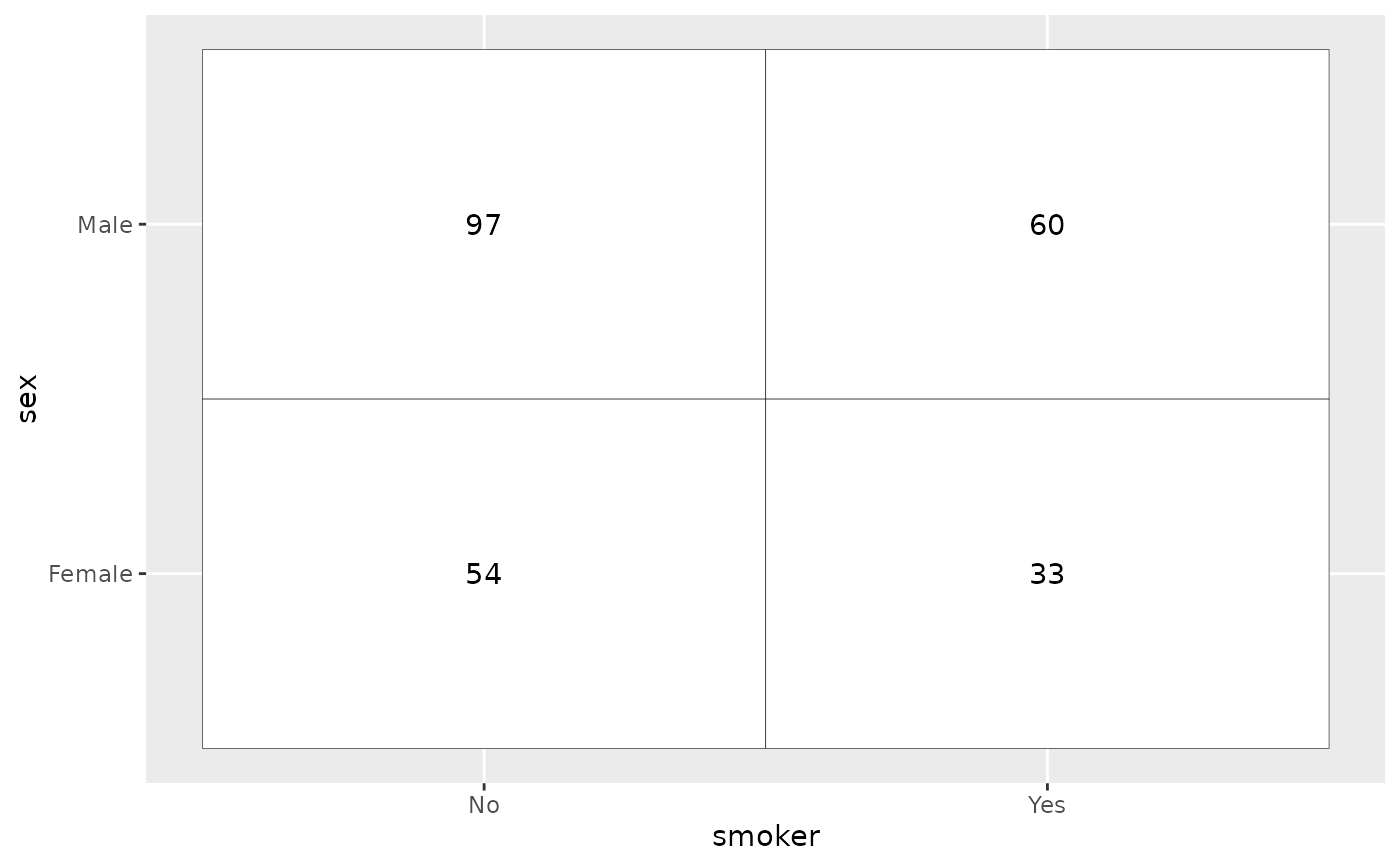# Use standardized residuals to fill table cells
p_(ggally_table(
as.data.frame(Titanic),
mapping = aes(
x = Class, y = Survived, weight = Freq,
fill = after_stat(std.resid),
label = scales::percent(after_stat(col.prop), accuracy = .1)
),
geom_tile_args = list(colour = "black")
) +
scale_fill_steps2(breaks = c(-3, -2, 2, 3), show.limits = TRUE))